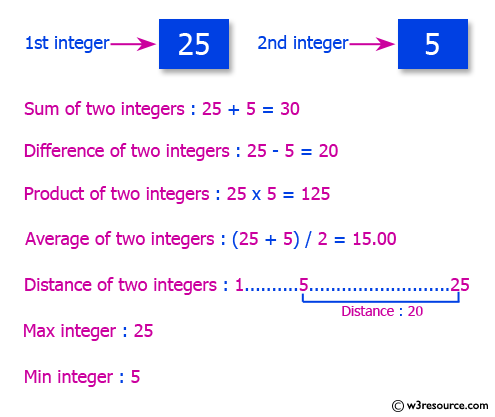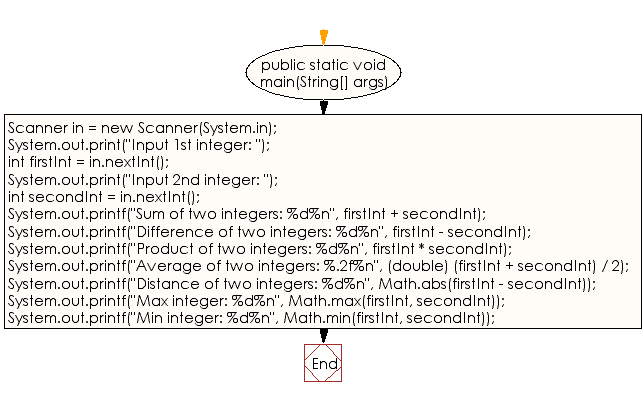﻿ Java exercises: Print the sum, difference, product, average, distance, maximum and minimum - w3resource Average of two integers: 15.00# Java Data Type Exercises: Print the sum, difference, product, average, distance, maximum and minimum

## Java Data Type: Exercise-9 with Solution

Write a Java program that accepts two integers and then prints the sum, the difference, the product, the average, the distance (the difference between integer), the maximum (the larger of the two integers), the minimum (smaller of the two integers).

Test Data
Input 1st integer: 25
Input 2nd integer: 5Sample Solution:

Java Code:

``````import java.util.Scanner;
public class Exercise9 {

public static void main(String[] args)
{
Scanner in = new Scanner(System.in);
System.out.print("Input 1st integer: ");
int firstInt = in.nextInt();
System.out.print("Input 2nd integer: ");
int secondInt = in.nextInt();

System.out.printf("Sum of two integers: %d%n", firstInt + secondInt);
System.out.printf("Difference of two integers: %d%n", firstInt - secondInt);
System.out.printf("Product of two integers: %d%n", firstInt * secondInt);
System.out.printf("Average of two integers: %.2f%n", (double) (firstInt + secondInt) / 2);
System.out.printf("Distance of two integers: %d%n", Math.abs(firstInt - secondInt));
System.out.printf("Max integer: %d%n", Math.max(firstInt, secondInt));
System.out.printf("Min integer: %d%n", Math.min(firstInt, secondInt));
}
}
```
```

Sample Output:

```Input 1st integer: 25
Input 2nd integer: 5
Sum of two integers: 30
Difference of two integers: 20
Product of two integers: 125
Average of two integers: 15.00
Distance of two integers: 20
Max integer: 25
Min integer: 5
```

Flowchart:Java Code Editor:

Improve this sample solution and post your code through Disqus

What is the difficulty level of this exercise?

﻿

## Java: Tips of the Day

Checks if a string is upper case:

```public static boolean isUpperCase(String input) {
return Objects.equals(input, input.toUpperCase());
}
```

Ref: https://bit.ly/39Hpo84